#### 相似文章# Thomas DeMark 对于技术分析做出的贡献

MetaTrader 4交易 | 12 九月 2016, 14:367 3752### 1. TD 点

Thomas DeMark 的第一项发明简化了寻找构建趋势线所需价格极值的过程。他决定使用日线图表来寻找最大价格的蜡烛条, 即高于前一天, 且高于随后 定义天数 (我将使用这个词来指代蜡烛条用于确定 TD 点存在)。如果满足该条件, 则可在图表上构建基于定义蜡烛条最大价格上的 TD 点。因此, 如果定义天数的最小值低于之前一天和随后几天的最小值, 则可在图表上构建基于定义蜡烛条最小价格上的 TD 点。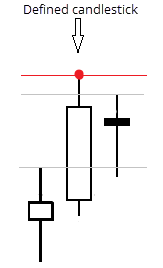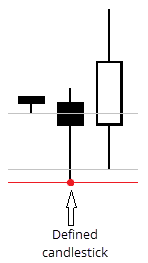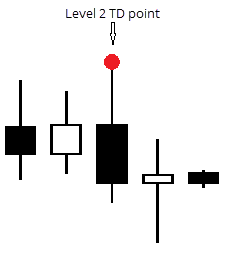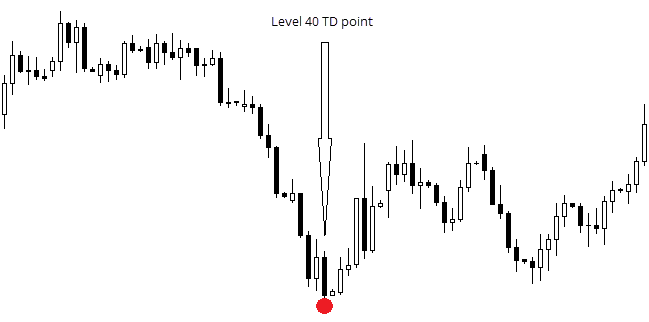### 2. TD 线

TD 点只是简单的单独极值。我们将需要两个点 (2 个最大值或 2 个最小值) 来建立一条趋势线。同时, Thomas DeMark 只使用 最后  两个明显的 TD 点。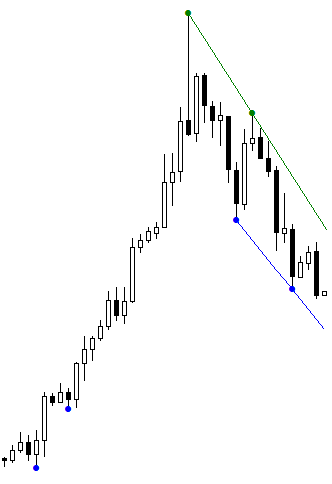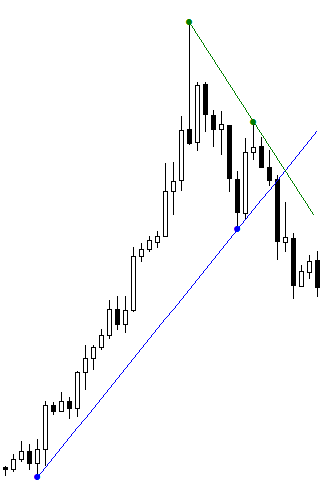Thomas DeMark 已经开发了 3 中价格项目可以直接链接到 TD 线, 我将在 "附加信息" 一节中简要触及该主题。

### 3.1. iTDDots

• 当挂载到图表上时, 基于存在的历史数据建立所有 TD 点。它们的级别由用户设置。
• 检查每根新蜡烛条建立新 TD 点的可能性, 如果出现则进一步处理。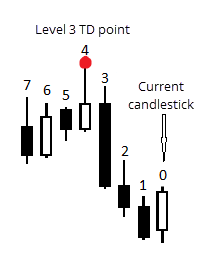以上插图显示如何通过指标按编号计算蜡烛条。它清晰地展示了其数额计算方法。

```#property indicator_chart_window        //在图表窗口里显示指标
#property indicator_buffers 2           //使用 2 个缓存区
#property indicator_plots   2           //2 个缓存区将用作显示
#property indicator_color1 clrGreen     //第一个缓存区的标准颜色
#property indicator_type1   DRAW_ARROW  //第一个缓存区的绘图类型
#property indicator_width1 2            //第一个缓存区显示的标准线宽
#property indicator_color2 clrBlue      //第二个缓存区的标准颜色
#property indicator_type2   DRAW_ARROW  //第二个缓存区的绘图类型
#property indicator_width2 2            //第二个缓存区显示的标准线宽

```

`input int Level = 1;`

```bool new_candle = true;
double  pdH,                    //定义蜡烛条的最大价格
pdL,                    //定义蜡烛条的最小价格
pricesH[],              //存储最大价格的数组
pricesL[];              //存储最小价格的数组
bool    DOTH,                   //显示一个点 (基于最大值)
DOTL;                   //显示一个点 (基于最小值)
double  UpDot[],                //基于最大值的绘图点缓存区数组
DownDot[];              //基于最小值的绘图点缓存区数组

```

```int OnInit()
{
ChartRedraw(0);                              //当切换时间帧时刷新图表
SetIndexBuffer(0, UpDot);
SetIndexBuffer(1, DownDot);
SetIndexEmptyValue(0,0.0);
SetIndexEmptyValue(1,0.0);
SetIndexArrow(0,159);                        //设置 Wingdings 字体的符号代码
SetIndexArrow(1,159);
SetIndexLabel(0, "TD " + Level + " High");   //这些名字将被显示在数据窗口
SetIndexLabel(1, "TD " + Level + " Low");
return(INIT_SUCCEEDED);
}

```

```void GetVariables(int start_candle, int level)
{
/*在此函数里, 指标从蜡烛条中收集建立 TD 点的信息。此处使用的所有变量已经全部声明为全局*/
pdH = iHigh(NULL, 0, start_candle + 1 + level);      //定义蜡烛的最高价
pdL = iLow(NULL, 0, start_candle + 1 + level);       //定义蜡烛的最低价

ArrayResize(pricesH, level * 2 + 1);                 //设置数组大小
ArrayResize(pricesL, level * 2 + 1);                 //

for (int i = level * 2; i >= 0; i--){                //收集数组中所需蜡烛的所有价格 (最大值和最小值)
pricesH[i] = iHigh(NULL, 0, start_candle + i + 1);
pricesL[i] = iLow(NULL, 0, start_candle + i + 1);
}

```

```int start()
{
int i = Bars - IndicatorCounted();                   //避免出现新蜡烛条时的重复计数
for (; i >= 0; i--)
{                                                  / 所/有 TD 点相关的活动在这里进行
DOTH = true;
DOTL = true;
GetVariables(i, Level);                           //获取当前价格值

for(int ii = 0; ii < ArraySize(pricesH); ii++)
{                                              //判断在此间隔是否为 TD 点
if (pdH < pricesH[ii]) DOTH = false;
if (pdL > pricesL[ii]) DOTL = false;
}

if(DOTH) UpDot[i + Level + 1] = pdH;              //如果是, 则其构造如下
if(DOTL) DownDot[i + Level + 1] = pdL;

if(UpDot[i + Level + 1] ==  UpDot[i + Level + 2]) UpDot[i + Level + 2] = 0;       //在此, 我涵盖了两根蜡烛条的情况
if(DownDot[i + Level + 1] ==  DownDot[i + Level + 2]) DownDot[i + Level + 2] = 0; //同时有最大值和最小值, 建立一个 TD 点
}                                                                                  //在最后一对蜡烛条
return(0);
}

```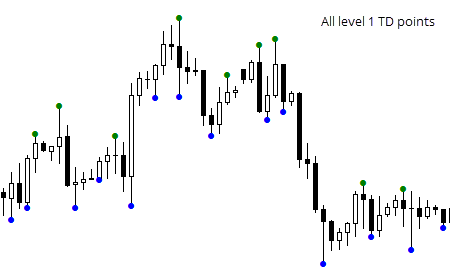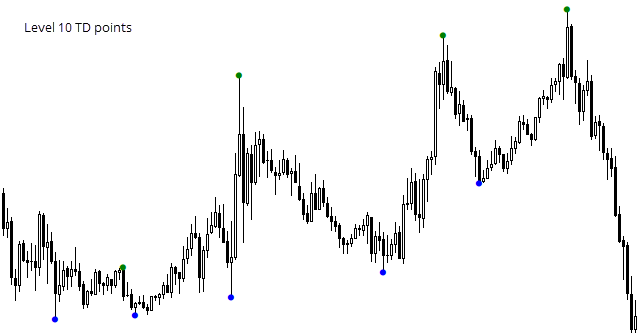### 3.2. iTDLines

k = (y2 - y1) / (x2 - x1),
b = (x2 * y1 - x1 * y2) / (x2 - x1),

x2 - 第二个点的蜡烛条号码,
y1 - 第一个点的价格,
y2 - 第二个点的价格。

```#property indicator_chart_window
#property indicator_buffers 2
#property indicator_plots 2

#property indicator_color1 clrGreen
#property indicator_color2 clrBlue

input int Level = 1;

double LU[], LD[];

//此变量用于最小值的计算。
datetime LastCount;

```

```void GetBK(double &Ub, double &Uk, double &Db, double &Dk, int &EndUN, int &EndDN)
{
double TDU[];
double TDD[];
int TDU_n[];
int TDD_n[];
ArrayResize(TDU, 2, 2);
ArrayResize(TDD, 2, 2);
ArrayResize(TDU_n, 2, 2);
ArrayResize(TDD_n, 2, 2);

```

```//接受支点的价格值, 以及自开头的蜡烛条号码
int Ui = 0;
int Di = 0;
for(int i = 0;; i++)
{
double current_bar_U = iCustom(NULL, 0, "TDDots", Level, 0, i);
double current_bar_D = iCustom(NULL, 0, "TDDots", Level, 1, i);

if(current_bar_U > 0 && Ui < 2)
{
TDU[Ui] = current_bar_U;   //价格
TDU_n[Ui] = i;             //号码
Ui++;
}
if(current_bar_D > 0 && Di < 2)
{
TDD[Di] = current_bar_D;
TDD_n[Di] = i;
Di++;
}
if(Ui == 2 && Di == 2) break;
}

```

```   Ub = ( (TDU_n * TDU) - (TDU * TDU_n) ) / ( TDU_n - TDU_n );
Uk = (TDU - TDU) / (TDU_n - TDU_n);

Db = ( (TDD_n * TDD) - (TDD_n * TDD) ) / ( TDD_n - TDD_n );
Dk = (TDD - TDD) / (TDD_n - TDD_n);

EndUN = TDU_n;
EndDN = TDD_n;
}

```

b = (x1 * y2 - x2 * y1) / (x1 - x2),
k = (y1 - y2) / (x1 - x2),

x2 - 第二个支点的蜡烛条号码,
y1 - 第一个点的价格,
y2 - 第二个点的价格。

```int OnInit()
{
SetIndexBuffer(0, LU);
SetIndexLabel(0, "TDLU");
SetIndexBuffer(1, LD);
SetIndexLabel(1, "TDLD");

SetIndexEmptyValue(0, 0);
SetIndexEmptyValue(1, 0);

LastCount = iTime(NULL, 0, 1);

return(INIT_SUCCEEDED);
}

```

```int start()
{
//新蜡烛或首次启动
if(iTime(NULL, 0, 0) != LastCount)
{
double Ub, Uk, Db, Dk;
int eUp, eDp;

GetBK(Ub, Uk, Db, Dk, eUp, eDp);

//删除旧数值
for(int i = 0; i < IndicatorCounted(); i++)
{
LU[i] = 0;
LD[i] = 0;
}

//建立新数值
for(i = 0; i <= eUp; i++)
{
LU[i] = Uk * i + Ub;
}

for(i = 0; i <= eDp; i++)
{
LD[i] = Dk * i + Db;
}

LastCount = iTime(NULL, 0, 0);
}

return 0;
}

```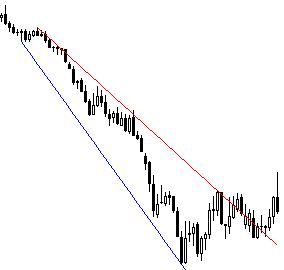### 3.3水平线指标

1. 指标获取由用户设定的确定级别 TD 点的 n 个数值
2. 计算这些点的平均价格
3. 基于它的水平线显示在图表上

2 个缓存区的数值和 3 个变量, 用户可输入:

```#property indicator_chart_window
#property indicator_buffers 2
#property indicator_plots   2
#property indicator_color1 clrGreen
#property indicator_type1   DRAW_LINE
#property indicator_width1 2
#property indicator_color2 clrBlue
#property indicator_type2   DRAW_LINE
#property indicator_width2 2

input int TDLevel = 1;       //点的级别
input int NumberOfDots = 3;  //点的数量
input double Delta = 0.001;  //两点之间最大距离

double TDLU[], TDLD[];

```

```int OnInit()
{
SetIndexBuffer(0,TDLU);
SetIndexBuffer(1,TDLD);
SetIndexEmptyValue(0,0.0);
SetIndexEmptyValue(1,0.0);
SetIndexLabel(0, "U HL");
SetIndexLabel(1, "D HL");
ObjectCreate(0, "U 水平级别", OBJ_HLINE, 0, iTime(NULL, 0, 0), 0);
ObjectCreate(0, "D 水平级别", OBJ_HLINE, 0, iTime(NULL, 0, 0), 0);
return(INIT_SUCCEEDED);
}

```

```double GetLevelPrice(int ud,int n,double delta,int level)
{
/* ud - 指标线型。0 - U, 其它值 - D。
n - 极点数量。
delta - 它们之间的最大距离
level - 点级别。*/

//准备保存极点价格的数组
double TDU[];
double TDD[];
ArrayResize(TDU,n,n);
ArrayResize(TDD,n,n);
ArrayInitialize(TDU,0);
ArrayInitialize(TDD,0);

//循环操作两次, 因为只存在两个数据缓存区
for(int Buffer=0; Buffer<2; Buffer++)
{
int N=0;
int Fails=0;
bool r=false;
for(int i=0; r==false; i++)
{
double d=iCustom(NULL,0,"TDDots",level,Buffer,i);
if(d>0)
{
if(N>0)
{
if(Buffer==0) double cp=TDU[N-1];
else cp=TDD[N-1];
if(MathAbs(d-cp)<=delta)
{
if(Buffer == 0)
TDU[N] = d;
else TDD[N]=d;
N++;
}
//如果距离太远, 则在错误中加 1
else
{
Fails++;
}
}
else
{
if(Buffer == 0)
TDU[N] = d;
else TDD[N]=d;

N++;
}
}
//如果错误太多, 循环终止
if(Fails>2 || N>n) r=true;
}
}

//获得平均值
double ATDU = 0;
double ATDD = 0;
N=0;
for(i=0; i<ArraySize(TDU); i++)
{
ATDU=ATDU+TDU[i];
if(TDU[i]==0)
{
i=ArraySize(TDU);
}
else
{
N++;
}
}
ATDU=ATDU/N;
N=0;
for(i=0; i<ArraySize(TDD); i++)
{
ATDD=ATDD+TDD[i];
if(TDD[i]==0)
{
i=ArraySize(TDD);
}
else
{
N++;
}
}
ATDD=ATDD/N;

//函数返回值
if(ud == 0) return ATDU;
else return ATDD;
}

```

每次新分时调用的函数里, 只简单地保留获取价格值并将它们分配给已创建对象:

```void start()
{
//从指标缓存区里删除前一根蜡烛条数值
TDLD = 0;
TDLU = 0;

TDLD = GetLevelPrice(1, TDLevel, Delta, NumberOfDots);
TDLU = GetLevelPrice(0, TDLevel, Delta, NumberOfDots);

//如果对象不知何故消失了
if(ObjectFind("U 水平级别") < 0)
{
ObjectCreate(0, "U 水平级别", OBJ_HLINE, 0, iTime(NULL, 0, 0), 0);
}
if(ObjectFind("D 水平级别") < 0)
{
ObjectCreate(0, "D 水平级别", OBJ_HLINE, 0, iTime(NULL, 0, 0), 0);
}

ObjectSetDouble(0, "U 水平级别", OBJPROP_PRICE, TDLU);
ObjectSetDouble(0, "D 水平级别", OBJPROP_PRICE, TDLD);
}

```

```void OnDeinit(const int reason)
{
if(!ObjectDelete("U 水平级别")) Print(GetLastError());
if(!ObjectDelete("D 水平级别")) Print(GetLastError());
}

```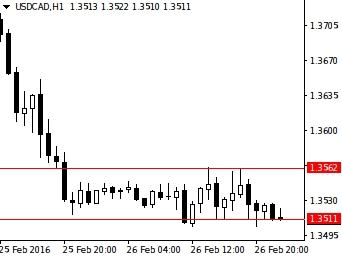### 4. 遵照水平线指标进行交易的智能程序

• 价格突破水平级别上边界
• 自当前水平级别上边界的价格增长 n 个点

• 价格突破水平级别下边界
• 自当前水平级别下边界的价格降低 n 个点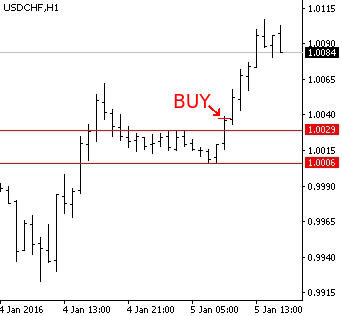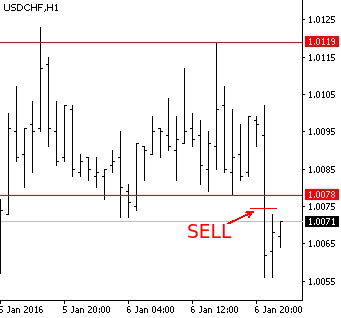```input int MagicNumber = 88341;      //智能交易程序开单时所用的魔幻数字
input int GL_TDLevel = 1;           //水平级别指标所用的 TD 点级别
input int GL_NumberOfDots = 3;      //水平级别指标所用的点数
input double S_ExtraPoints = 0.0001;//附加点数
input double GL_Delta = 0.001;      //水平级别指标认可的 TD 点距离
input int StopLoss = 50;            //止损位
input double Lot = 0.01;            //手数大小

```

```int GetSignal(string symbol,int TF,int TDLevel,int NumberOfDots,int Delta,double ExtraPoints)
{
//建立 TD 点上边界的价格值
double UL=iCustom(symbol,TF,"iglevels",GL_TDLevel,GL_NumberOfDots,GL_Delta,0,0)+ExtraPoints;
//...TD 点的下边界
double DL=iCustom(symbol,TF,"iglevels",GL_TDLevel,GL_NumberOfDots,GL_Delta,1,0)-ExtraPoints;

if(Bid<DL && iLow(symbol,TF,1)>DL)
{
return 1;
}
else
{
{
return 0;
}
else
{
return -1;
}
}
}

```

```int Signal = -1;          //当前信号
datetime LastOrder;       //最后执行交易的日期。为避免在一根蜡烛条上重复开单的情况, 这是必需的。

```

```int OnInit()
{
LastOrder = iTime(NULL, 0, 1);
return(INIT_SUCCEEDED);
}

```

```void OnTick()
{
bool order_is_open=false;
//搜索已开订单
for(int i=0; i<OrdersTotal(); i++)
{
if(!OrderSelect(i,SELECT_BY_POS)) Print(GetLastError());

if(OrderMagicNumber()==MagicNumber)
{
order_is_open=true;
break;
}
}

//获取当前信号
Signal=GetSignal(Symbol(),0,GL_TDLevel,GL_NumberOfDots,GL_Delta,S_ExtraPoints);

//计算止损大小
double tsl=NormalizeDouble(StopLoss*MathPow(10,-Digits),Digits);

if(order_is_open==true)
{
//计算止损价格

if(OrderType()==0 && OrderStopLoss()<p)
{
if(!OrderModify(OrderTicket(),OrderOpenPrice(),p,0,0)) Print(GetLastError());
}
if(OrderType()==1 && OrderStopLoss()>p)
{
if(!OrderModify(OrderTicket(),OrderOpenPrice(),p,0,0)) Print(GetLastError());
}
}
//如果没有订单
if(order_is_open==false)
{
//如果在当前蜡烛条尚未开单
if(iTime(NULL,0,0)!=LastOrder)
{
//买入
if(Signal==0)
{
LastOrder=iTime(NULL,0,0);
}
//卖出
if(Signal==1)
{
LastOrder=iTime(NULL,0,0);
}
}
}
}

```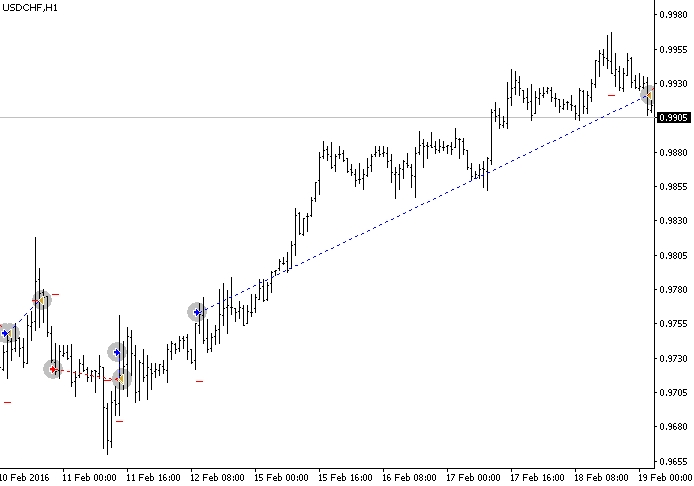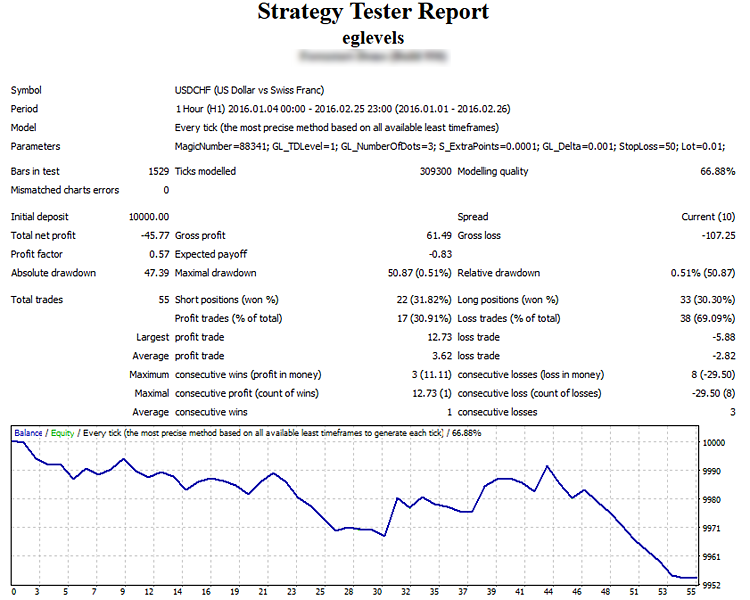### 5. 依据 TD 线进行交易的智能程序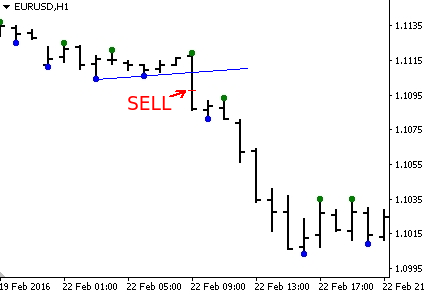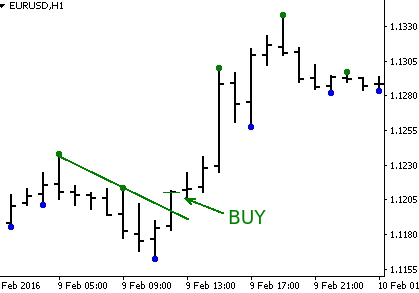The position is accompanied with a fixed size Trailing Stop. 平仓仅由止损执行。

```input int Level=1;                 //TD 线级别
input double Lot=0.01;             //手数大小
input int Magic=88342;             //订单的魔幻数字
input int Stop=50;                 //止损的点数
input int Bars_To_Open_New_Order=2;//开单的柱线间隔

datetime LastBar;

```

EA 在 OnInit 函数里为 LastBar 和 Trade_is_allowed 变量赋值:

```int OnInit()
{
LastBar=iTime(NULL,0,1);

return(INIT_SUCCEEDED);
}

```

```int GetSignal()
{
//如果新蜡烛条生成
if(LastBar!=iTime(NULL,0,0))
{
double DU = iCustom(NULL, 0, "itdlines", Level, 0, 0);
double DD = iCustom(NULL, 0, "itdlines", Level, 1, 0);
double DU1 = iCustom(NULL, 0, "itdlines", Level, 0, 1);
double DD1 = iCustom(NULL, 0, "itdlines", Level, 1, 1);
}

//上边线突破 --> 买入
{
return 0;
}
else
{
//下边线突破 --> 卖出
{
return 1;
}
//无突破 --> 忽略信号
else
{
return -1;
}
}

return -1;
}

```

```void OnTick()
{
int signal=GetSignal();
bool order_is_open=false;

//搜索已开单
for(int i=0; i<OrdersTotal(); i++)
{
if(!OrderSelect(i,SELECT_BY_POS)) Print(GetLastError());

//通过魔幻数字
if(OrderMagicNumber()==Magic)
{
order_is_open=true;
i=OrdersTotal();
}
}

//止损距离
double stop=Stop*MathPow(10,-Digits);
//如果订单以开仓
if(order_is_open==true)
{
//检查移动止损的可能性

//止损价位
double order_stop=NormalizeDouble(OrderStopLoss(),Digits);

//如果买入订单
if(OrderType()==0)
{
{
}
}
//如果卖出订单
if(OrderType()==1)
{
if(order_stop>NormalizeDouble(Bid+stop,Digits))
{
if(!OrderModify(OrderTicket(),OrderOpenPrice(),NormalizeDouble(Bid+stop,Digits),0,0))Print(GetLastError());
}
}
}
//如果尚未开单
else
{
{
if(signal==0)
{
}
if(signal==1)
{
if(!OrderSend(NULL,signal,Lot,Bid,5,Bid+stop,0,NULL,Magic)) Print(GetLastError());
}

}
}
}

```

EA 交易依据: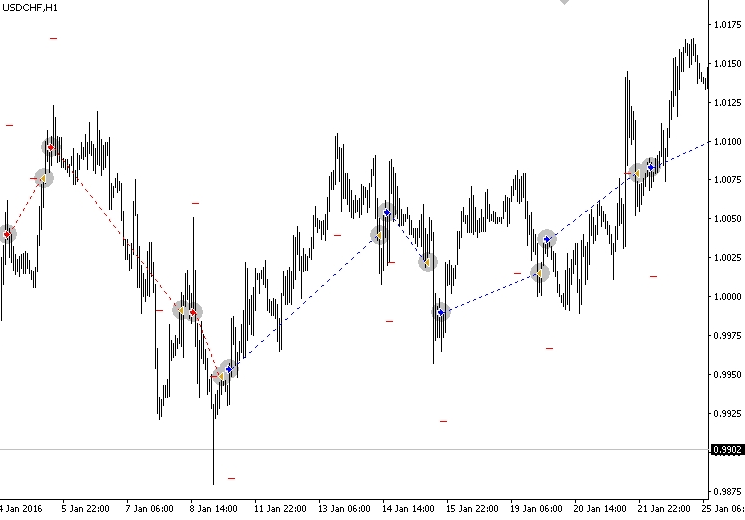EA 在长线行情下交易良好是显而易见的, 不过, 犬牙交错的行情下会产生一定数量的假信号并导致交易无利可图。EA 的测试结果如下: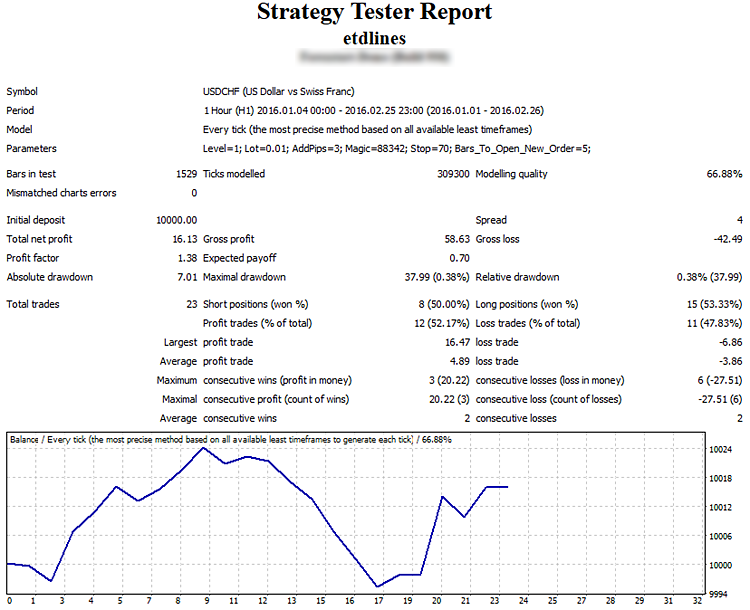### 结论

eglevels.mq4 (7.22 KB)
etdlines.mq4 (7.92 KB)
iglevels.mq4 (9.15 KB)
itdlines.mq4 (6.57 KB)
itddots.mq4 (5.83 KB)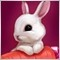| 15 9月 2016 在 06:35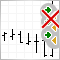交易机器人的虚假触发保护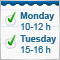使用文本文件保存智能交易程序, 指标和脚本的输入参数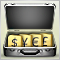在 MetaTrader 4 中的投资组合交易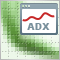以横盘和趋势行情为例强化策略测试器的指标优化